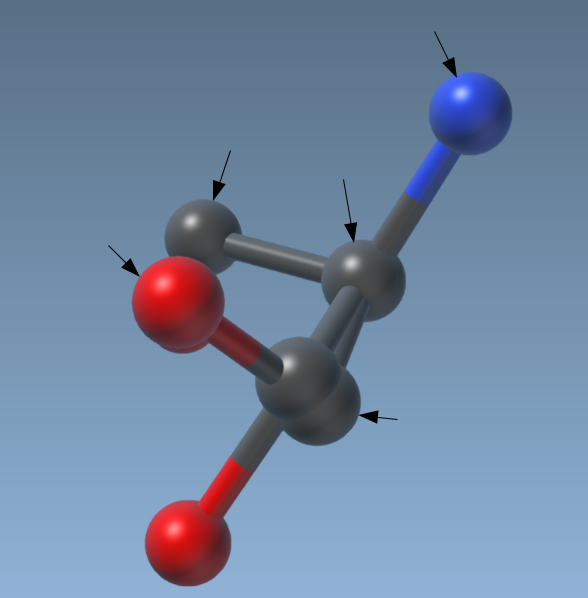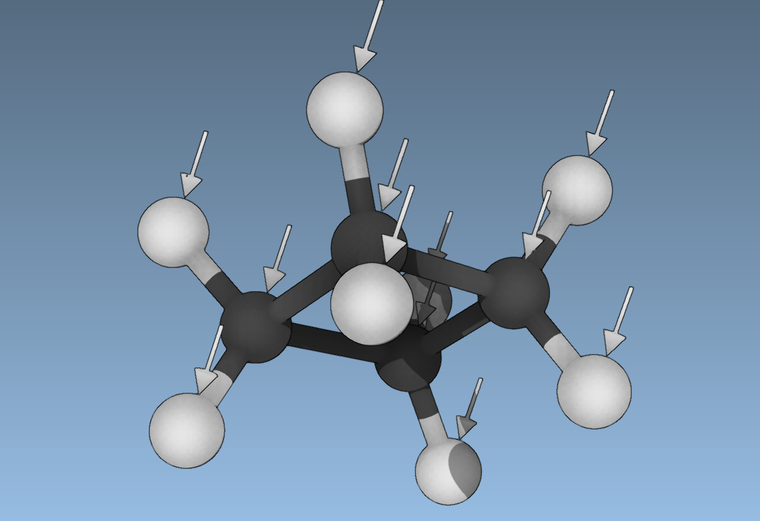# Drawing arrows on atoms

• Hi,
I would like to draw arrows on atoms in order to indicate the user how to apply forces on atoms. Is it possible to do in SAMSON? If yes, would anyone mind helping me do it, please? Here is what I would like to get as result in SAMSON if possible:• @piriziwè For that you can use function SAMSON::displayCylinders.
So, for example, one cylinder for the line and one cylinder for the arrow head with one of the radiuses set to zero. A line may also be drawn using SAMSON::displayLines.

• Hi @Piriziwè ,

Here is an example code that you may use and that puts vertical arrows on every atom.

``````
void SEArrowDrawerVisualModel::display() {
// NodeIndexer that will receive the atoms
SBNodeIndexer nodes;
SAMSON::getActiveDocument()->getNodes(nodes, SBNode::IsType(Type::Atom));
// put all the atoms in the nodeIndexer

//3d Arrows example
unsigned int nCylinders = 2 * nodes.size(); // we will need 2 cylinders by arrow, one for the head, one for the stick
unsigned int nPositions = 4 * nodes.size(); // 3 position by arrow, the two end points and the middle
unsigned int* indexData = new unsigned int[2 * nCylinders]; // this should be of size 2* cylinder, it will say for each end point at which position it should be
float *positionData = new float[3 * nPositions]; // all the coordinates X Y Z of each positions,
float *radiusData = new float[2 * nCylinders]; // the radius of the two end points of the cylinder can be different to make a cone
unsigned int *capData = new unsigned int[2 * nCylinders]; // controls if the cylinders are closed or open
float *colorData = new float[4 * 2 * nCylinders]; // the ARGB code for each end point of a cylinder
unsigned int *flagData = new unsigned int[2 * nCylinders]; // controls if the cylinder is highlighted, selected
int atomCounter = 0;
SB_FOR(SBNode* n, nodes) {
SBAtom* atom = (SBAtom*) n;

SBVector3 arrowDirection(1, 0, 0);
SBQuantity::length arrowLength(60);

// give the position of the cone that is the head of the arrow
positionData[atomCounter * 12 + 0] = (atom->getPosition().v + arrowDirection.v * SAMSON::getAtomRadius()).getValue();
positionData[atomCounter * 12 + 1] = (atom->getPosition().v + arrowDirection.v * SAMSON::getAtomRadius()).getValue();
positionData[atomCounter * 12 + 2] = (atom->getPosition().v + arrowDirection.v * SAMSON::getAtomRadius()).getValue();
positionData[atomCounter * 12 + 3] = (atom->getPosition().v + arrowDirection.v * (SAMSON::getAtomRadius() + 0.3 * arrowLength)).getValue();
positionData[atomCounter * 12 + 4] = (atom->getPosition().v + arrowDirection.v * (SAMSON::getAtomRadius() + 0.3 * arrowLength)).getValue();
positionData[atomCounter * 12 + 5] = (atom->getPosition().v + arrowDirection.v * (SAMSON::getAtomRadius() + 0.3 * arrowLength)).getValue();
// radii of the cone are 0 on the pointed size and 0 on the other side
radiusData[atomCounter * 4 + 0] = 0;
radiusData[atomCounter * 4 + 1] = 10;

// give the position of the cylinder that is the tail of the arrow
positionData[atomCounter * 12 + 6] = (atom->getPosition().v + arrowDirection.v * (SAMSON::getAtomRadius() + 0.3 * arrowLength)).getValue();
positionData[atomCounter * 12 + 7] = (atom->getPosition().v + arrowDirection.v * (SAMSON::getAtomRadius() + 0.3 * arrowLength)).getValue();
positionData[atomCounter * 12 + 8] = (atom->getPosition().v + arrowDirection.v * (SAMSON::getAtomRadius() + 0.3 * arrowLength)).getValue();
positionData[atomCounter * 12 + 9] = (atom->getPosition().v + arrowDirection.v * (SAMSON::getAtomRadius() + arrowLength)).getValue();
positionData[atomCounter * 12 + 10] = (atom->getPosition().v + arrowDirection.v * (SAMSON::getAtomRadius() + arrowLength)).getValue();
positionData[atomCounter * 12 + 11] = (atom->getPosition().v + arrowDirection.v * (SAMSON::getAtomRadius() + arrowLength)).getValue();
// radius of the tail is 2 everywhere
radiusData[atomCounter * 4 + 2] = 2;
radiusData[atomCounter * 4 + 3] = 2;

indexData[atomCounter * 4 + 0] = 4 * atomCounter + 0;
indexData[atomCounter * 4 + 1] = 4 * atomCounter + 1;
indexData[atomCounter * 4 + 2] = 4 * atomCounter + 2;
indexData[atomCounter * 4 + 3] = 4 * atomCounter + 3;

capData[atomCounter * 4 + 0] = 1;
capData[atomCounter * 4 + 1] = 1;
capData[atomCounter * 4 + 2] = 1;
capData[atomCounter * 4 + 3] = 1;

colorData[atomCounter * 16 + 0 ] = 1;
colorData[atomCounter * 16 + 1 ] = 1;
colorData[atomCounter * 16 + 2 ] = 1;
colorData[atomCounter * 16 + 3 ] = 1;
colorData[atomCounter * 16 + 4 ] = 1;
colorData[atomCounter * 16 + 5 ] = 1;
colorData[atomCounter * 16 + 6 ] = 1;
colorData[atomCounter * 16 + 7 ] = 1;
colorData[atomCounter * 16 + 8 ] = 1;
colorData[atomCounter * 16 + 9 ] = 1;
colorData[atomCounter * 16 + 10] = 1;
colorData[atomCounter * 16 + 11] = 1;
colorData[atomCounter * 16 + 12] = 1;
colorData[atomCounter * 16 + 13] = 1;
colorData[atomCounter * 16 + 14] = 1;
colorData[atomCounter * 16 + 15] = 1;

unsigned int flag = (atom->isSelected() ? 1 : 0) + (atom->isHighlighted() ? 2 : 0);
flagData[atomCounter * 4 + 0] = flag;
flagData[atomCounter * 4 + 1] = flag;
flagData[atomCounter * 4 + 2] = flag;
flagData[atomCounter * 4 + 3] = flag;

atomCounter++;
}

SAMSON::displayCylinders(nCylinders, nPositions, indexData, positionData, radiusData, capData, colorData, flagData);

delete[] indexData;
delete[] capData;
delete[] colorData;
delete[] flagData;

}
``````• @guillaume Hi,
Thank you very much for this example. I will try it and send you a feedback.

• Hi @guillaume, I tried your example above and it works correctly. But now I would like to call the visual model by code (on click event of a button) instead of doing it from SAMSON "Visualization->Add visual model" menu. I tried like adding a structural model but it does not work. Can you help me, please?

Thank you.

• @Guillaume, It's done :) Thanks

• I'm glad you managed to do it, here is my solution if other people need it:

``````
SEMyVisualModel* vm = new SEMyVisualModel();// create your visual model
SAMSON::beginHolding("Create visual model"); // this is to have this action undoable
SAMSON::hold(vm); // Give the property of the object to SAMSON, you will not have to delete it when it will be removed
vm->create();
SAMSON::endHolding();
``````

• Hi @guillaume, please, I am facing a issue. Which paramters can I modify in order to get horizontal arrows or other directions arrows, please? Thank you in advance.

• Hi @piriziwè ,

``````		SBVector3 arrowDirection(1, 0, 0);
SBQuantity::length arrowLength(60);

// give the position of the cone that is the head of the arrow
positionData[atomCounter * 12 + 0] = (atom->getPosition().v + arrowDirection.v * SAMSON::getAtomRadius()).getValue();
positionData[atomCounter * 12 + 1] = (atom->getPosition().v + arrowDirection.v * SAMSON::getAtomRadius()).getValue();
positionData[atomCounter * 12 + 2] = (atom->getPosition().v + arrowDirection.v * SAMSON::getAtomRadius()).getValue();
positionData[atomCounter * 12 + 3] = (atom->getPosition().v + arrowDirection.v * (SAMSON::getAtomRadius() + 0.3 * arrowLength)).getValue();
positionData[atomCounter * 12 + 4] = (atom->getPosition().v + arrowDirection.v * (SAMSON::getAtomRadius() + 0.3 * arrowLength)).getValue();
positionData[atomCounter * 12 + 5] = (atom->getPosition().v + arrowDirection.v * (SAMSON::getAtomRadius() + 0.3 * arrowLength)).getValue();
// radii of the cone are 0 on the pointed size and 0 on the other side
radiusData[atomCounter * 4 + 0] = 0;
radiusData[atomCounter * 4 + 1] = 10;

// give the position of the cylinder that is the tail of the arrow
positionData[atomCounter * 12 + 6] = (atom->getPosition().v + arrowDirection.v * (SAMSON::getAtomRadius() + 0.3 * arrowLength)).getValue();
positionData[atomCounter * 12 + 7] = (atom->getPosition().v + arrowDirection.v * (SAMSON::getAtomRadius() + 0.3 * arrowLength)).getValue();
positionData[atomCounter * 12 + 8] = (atom->getPosition().v + arrowDirection.v * (SAMSON::getAtomRadius() + 0.3 * arrowLength)).getValue();
positionData[atomCounter * 12 + 9] = (atom->getPosition().v + arrowDirection.v * (SAMSON::getAtomRadius() + arrowLength)).getValue();
positionData[atomCounter * 12 + 10] = (atom->getPosition().v + arrowDirection.v * (SAMSON::getAtomRadius() + arrowLength)).getValue();
positionData[atomCounter * 12 + 11] = (atom->getPosition().v + arrowDirection.v * (SAMSON::getAtomRadius() + arrowLength)).getValue();
// radius of the tail is 2 everywhere
radiusData[atomCounter * 4 + 2] = 2;
radiusData[atomCounter * 4 + 3] = 2;

``````

I did not detailed this par much, you can change the value of arrow direction to get the direction you want for the arrow. It can even be a different value for different atoms., I will update the first post as well

• Thank you @guillaume. I will try it and see. Have a nice weekend

• Hi everybody, all my problems with arrows are solved. Thanks very much for your help.

Looks like your connection to SAMSON Connect - Forum was lost, please wait while we try to reconnect.